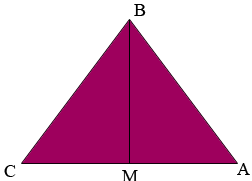SEARCH HOMEMath Central Quandaries & QueriesQuestion from Reagan: I need to find the height of an isosceles triangle with a base of 6 and sides of 4 units. How do I find it?Hi Reagan,In my diagram $M$ is the midpoint of the base. Triangles $ABM$ and $BCM$ are congruent. Do you se why? Thus angles $BMA$ and $CMB$ are congruent. The sum of heir measures is $180^o$ so each of them is a right angle. Now you can use Pythagoras Theorem.

PennyMath Central is supported by the University of Regina and The Pacific Institute for the Mathematical Sciences.